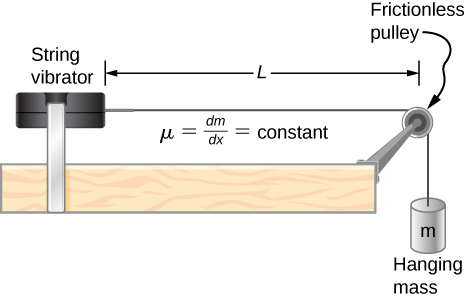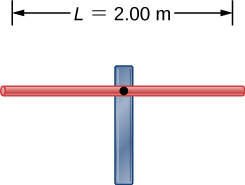# 16.6 Standing waves and resonance  (Page 7/17)

 Page 7 / 17

Why do roofs of gymnasiums and churches seem to fail more than family homes when an earthquake occurs?

Wine glasses can be set into resonance by moistening your finger and rubbing it around the rim of the glass. Why?

Energy is supplied to the glass by the work done by the force of your finger on the glass. When supplied at the right frequency, standing waves form. The glass resonates and the vibrations produce sound.

Air conditioning units are sometimes placed on the roof of homes in the city. Occasionally, the air conditioners cause an undesirable hum throughout the upper floors of the homes. Why does this happen? What can be done to reduce the hum?

Consider a standing wave modeled as $y\left(x,t\right)=4.00\phantom{\rule{0.2em}{0ex}}\text{cm}\phantom{\rule{0.2em}{0ex}}\text{sin}\left(3\phantom{\rule{0.2em}{0ex}}{\text{m}}^{-1}x\right)\text{cos}\left(4\phantom{\rule{0.2em}{0ex}}{\text{s}}^{-1}t\right).$ Is there a node or an antinode at $x=0.00\phantom{\rule{0.2em}{0ex}}\text{m}?$ What about a standing wave modeled as $y\left(x,t\right)=4.00\phantom{\rule{0.2em}{0ex}}\text{cm}\phantom{\rule{0.2em}{0ex}}\text{sin}\left(3\phantom{\rule{0.2em}{0ex}}{\text{m}}^{-1}x+\frac{\pi }{2}\right)\text{cos}\left(4\phantom{\rule{0.2em}{0ex}}{\text{s}}^{-1}t\right)?$ Is there a node or an antinode at the $x=0.00\phantom{\rule{0.2em}{0ex}}\text{m}$ position?

For the equation $y\left(x,t\right)=4.00\phantom{\rule{0.2em}{0ex}}\text{cm}\phantom{\rule{0.2em}{0ex}}\text{sin}\left(3\phantom{\rule{0.2em}{0ex}}{\text{m}}^{-1}x\right)\text{cos}\left(4\phantom{\rule{0.2em}{0ex}}{\text{s}}^{-1}t\right),$ there is a node because when $x=0.00\phantom{\rule{0.2em}{0ex}}\text{m}$ , $\text{sin}\left(3\phantom{\rule{0.2em}{0ex}}{\text{m}}^{-1}\left(0.00\phantom{\rule{0.2em}{0ex}}\text{m}\right)\right)=0.00,$ so $y\left(0.00\phantom{\rule{0.2em}{0ex}}\text{m},t\right)=0.00\phantom{\rule{0.2em}{0ex}}\text{m}$ for all time. For the equation $y\left(x,t\right)=4.00\phantom{\rule{0.2em}{0ex}}\text{cm}\phantom{\rule{0.2em}{0ex}}\text{sin}\left(3\phantom{\rule{0.2em}{0ex}}{\text{m}}^{-1}x+\frac{\pi }{2}\right)\text{cos}\left(4\phantom{\rule{0.2em}{0ex}}{\text{s}}^{-1}t\right),$ there is an antinode because when $x=0.00\phantom{\rule{0.2em}{0ex}}\text{m}$ , $\text{sin}\left(3\phantom{\rule{0.2em}{0ex}}{\text{m}}^{-1}\left(0.00\phantom{\rule{0.2em}{0ex}}\text{m}\right)+\frac{\pi }{2}\right)=+1.00$ , so $y\left(0.00\phantom{\rule{0.2em}{0ex}}\text{m},t\right)$ oscillates between + A and − A as the cosine term oscillates between +1 and -1.

## Problems

A wave traveling on a Slinky® that is stretched to 4 m takes 2.4 s to travel the length of the Slinky and back again. (a) What is the speed of the wave? (b) Using the same Slinky stretched to the same length, a standing wave is created which consists of three antinodes and four nodes. At what frequency must the Slinky be oscillating?

A 2-m long string is stretched between two supports with a tension that produces a wave speed equal to ${v}_{w}=50.00\phantom{\rule{0.2em}{0ex}}\text{m/s}.$ What are the wavelength and frequency of the first three modes that resonate on the string?

$\begin{array}{}\\ \\ {\lambda }_{n}=\frac{2.00}{n}L,\phantom{\rule{1em}{0ex}}{f}_{n}=\frac{v}{{\lambda }_{n}}\hfill \\ {\lambda }_{1}=4.00\phantom{\rule{0.2em}{0ex}}\text{m},\phantom{\rule{1em}{0ex}}{f}_{1}=12.5\phantom{\rule{0.2em}{0ex}}\text{Hz}\hfill \\ {\lambda }_{2}=2.00\phantom{\rule{0.2em}{0ex}}\text{m},\phantom{\rule{1em}{0ex}}{f}_{2}=25.00\phantom{\rule{0.2em}{0ex}}\text{Hz}\hfill \\ {\lambda }_{3}=1.33\phantom{\rule{0.2em}{0ex}}\text{m},\phantom{\rule{1em}{0ex}}{f}_{3}=37.59\phantom{\rule{0.2em}{0ex}}\text{Hz}\hfill \end{array}$

Consider the experimental setup shown below. The length of the string between the string vibrator and the pulley is $L=1.00\phantom{\rule{0.2em}{0ex}}\text{m}.$ The linear density of the string is $\mu =0.006\phantom{\rule{0.2em}{0ex}}\text{kg/m}.$ The string vibrator can oscillate at any frequency. The hanging mass is 2.00 kg. (a)What are the wavelength and frequency of $n=6$ mode? (b) The string oscillates the air around the string. What is the wavelength of the sound if the speed of the sound is ${v}_{s}=343.00\phantom{\rule{0.2em}{0ex}}\text{m/s?}$A cable with a linear density of $\mu =0.2\phantom{\rule{0.2em}{0ex}}\text{kg/m}$ is hung from telephone poles. The tension in the cable is 500.00 N. The distance between poles is 20 meters. The wind blows across the line, causing the cable resonate. A standing waves pattern is produced that has 4.5 wavelengths between the two poles. The air temperature is $T=20\text{°}\text{C}.$ What are the frequency and wavelength of the hum?

$\begin{array}{}\\ \\ v=158.11\phantom{\rule{0.2em}{0ex}}\text{m/s,}\phantom{\rule{1em}{0ex}}\lambda =4.44\phantom{\rule{0.2em}{0ex}}\text{m,}\phantom{\rule{1em}{0ex}}f=35.61\phantom{\rule{0.2em}{0ex}}\text{Hz}\hfill \\ {\lambda }_{s}=9.63\phantom{\rule{0.2em}{0ex}}\text{m}\hfill \end{array}$

Consider a rod of length L , mounted in the center to a support. A node must exist where the rod is mounted on a support, as shown below. Draw the first two normal modes of the rod as it is driven into resonance. Label the wavelength and the frequency required to drive the rod into resonance.Consider two wave functions $y\left(x,t\right)=0.30\phantom{\rule{0.2em}{0ex}}\text{cm}\phantom{\rule{0.2em}{0ex}}\text{sin}\left(3\phantom{\rule{0.2em}{0ex}}{\text{m}}^{-1}x-4\phantom{\rule{0.2em}{0ex}}{\text{s}}^{-1}t\right)$ and $y\left(x,t\right)=0.30\phantom{\rule{0.2em}{0ex}}\text{cm}\phantom{\rule{0.2em}{0ex}}\text{sin}\left(3\phantom{\rule{0.2em}{0ex}}{\text{m}}^{-1}x+4\phantom{\rule{0.2em}{0ex}}{\text{s}}^{-1}t\right)$ . Write a wave function for the resulting standing wave.

$y\left(x,t\right)=\left[0.60\phantom{\rule{0.2em}{0ex}}\text{cm}\phantom{\rule{0.2em}{0ex}}\text{sin}\left(3\phantom{\rule{0.2em}{0ex}}{\text{m}}^{-1}x\right)\right]\text{cos}\left(4\phantom{\rule{0.2em}{0ex}}{\text{s}}^{-1}t\right)$

explain equilibrium of a body
Hi all, love you all!!!
Cool
Debabrata
How does resonance occur
plzz explanation of the equilibrium of a body
what is quantam
quantum is a division of mechanics
Baje
what is friction
a force act by surface between two bodies whose are always oppose the relative motion .....
Raghav
when two rough bodies are placed in contact and try to slip each other ... than a force act them and it's ippse the relative motion between them
Raghav
thats friction force and roughnes of both bodies is define friction of surface
Raghav
what is a progressive wave
What is the wake for therapist
can u like explain your question with clear detail
Chikamso
who would teach me vectors?
what's chemistry
branch of science dt deals with the study of physical properties of matter and it's particulate nature
Josiah
Good
Daniel
actually
Nathz
Y acctually do u hav ur way of defining it? just bring ur iwn idear
Daniel
well, it deals with the weight of substances and reaction behind them as well as the behavior
Josiah
buh hope Esther, we've answered ur question
Josiah
what's ohms law
CHIJIOKE
ohms law states that, the current flowing through an electric circuit is directly proportional to the potential difference, provided temperature and pressure are kept constant
Josiah
what is sound
James
ohms law states that the resistance of a material is directly proportional to the potential difference between two points on that material, if temperature and other physical conditions become constant
Chikamso
How do I access the MCQ
As I think the best is, first select the easiest questions for you .and then you can answer the remaining questions.
lasitha
I mean I'm unable to view it
Abraham
when I click on it, it doesn't respond
Abraham
ohhh,try again and again ,It will be showed
lasitha
okay
Abraham
what is centripetal force
هي قوة ناتجة من الحركة الدائرية ويكون اتجاهها إلى المركز دائماً
meaning of vector quantity
vector quantity is any quantity that has both magnitude in terms of number (units) and direction in terms of viewing the quantity from an origin using angles (degree) or (NEWS) method
LEWIS
vector quantity is physical quantity has magnitude and direction
vector is a quantity that is use in measuring size of physical properties and their direction
Bitrus
what difference and similarities between work,force,energy and power?
Anes
power
mehreen
power
saba
enery is the ability to do work. work is job done, force is a pull or push. power has to do with potential. they belong to different categories which include heat energy, electricity.
Andrew
force refers to a push or pull... energy refers to work done while power is work done per unit time
Shane
mathematically express angular velocity and angular acceleration
it depends on the direction. an angular velocity will be linear and angular acceleration will be an angle of elevation.
Andrew
The sonic range finder discussed in the preceding question often needs to be calibrated. During the calibration, the software asks for the room temperature. Why do you suppose the room temperature is required?
Suppose a bat uses sound echoes to locate its insect prey, 3.00 m away. (See [link] .) (a) Calculate the echo times for temperatures of 5.00°C5.00°C and 35.0°C.35.0°C. (b) What percent uncertainty does this cause for the bat in locating the insect? (c) Discuss the significance of this uncertainty an
Shaina
give a reason why musicians commonly bring their wind instruments to room temperature before playing them.
Shaina
The ear canal resonates like a tube closed at one end. (See [link]Figure 17_03_HumEar[/link].) If ear canals range in length from 1.80 to 2.60 cm in an average population, what is the range of fundamental resonant frequencies? Take air temperature to be 37.0°C,37.0°C, which is the same as body tempe
Shaina
By what fraction will the frequencies produced by a wind instrument change when air temperature goes from 10.0°C10.0°C to 30.0°C30.0°C ? That is, find the ratio of the frequencies at those temperatures.
ShainaByBy Anindyo MukhopadhyayByBy